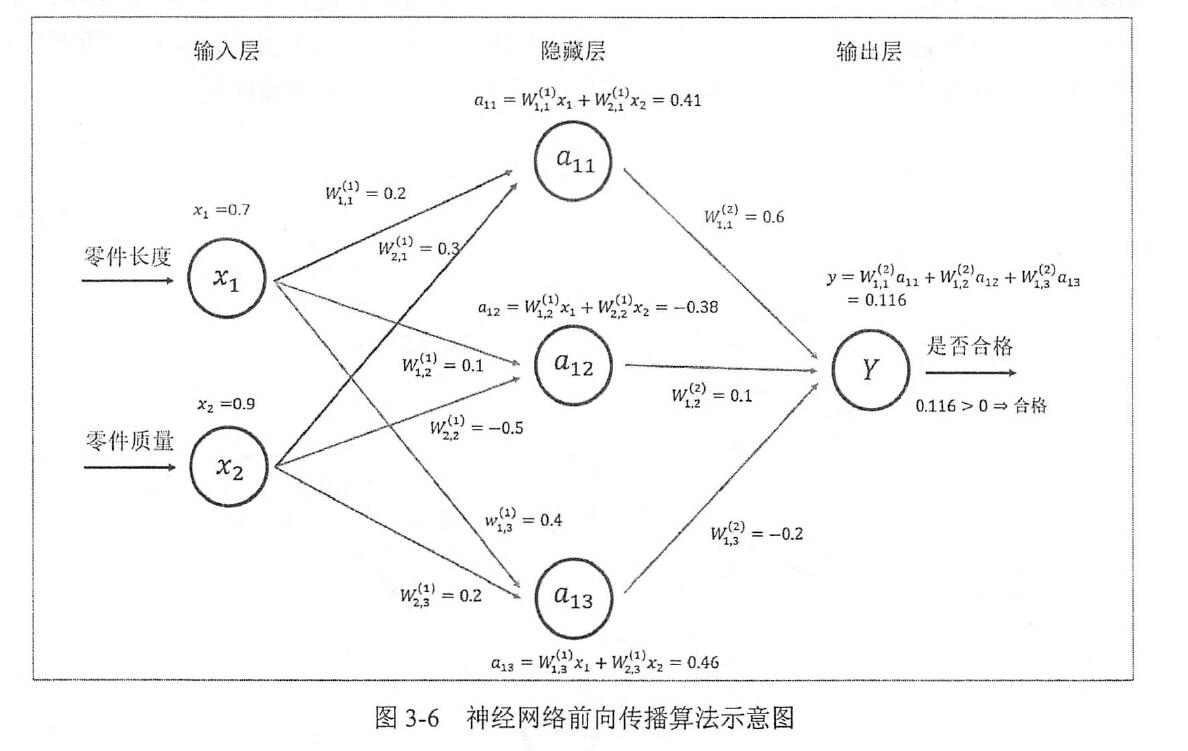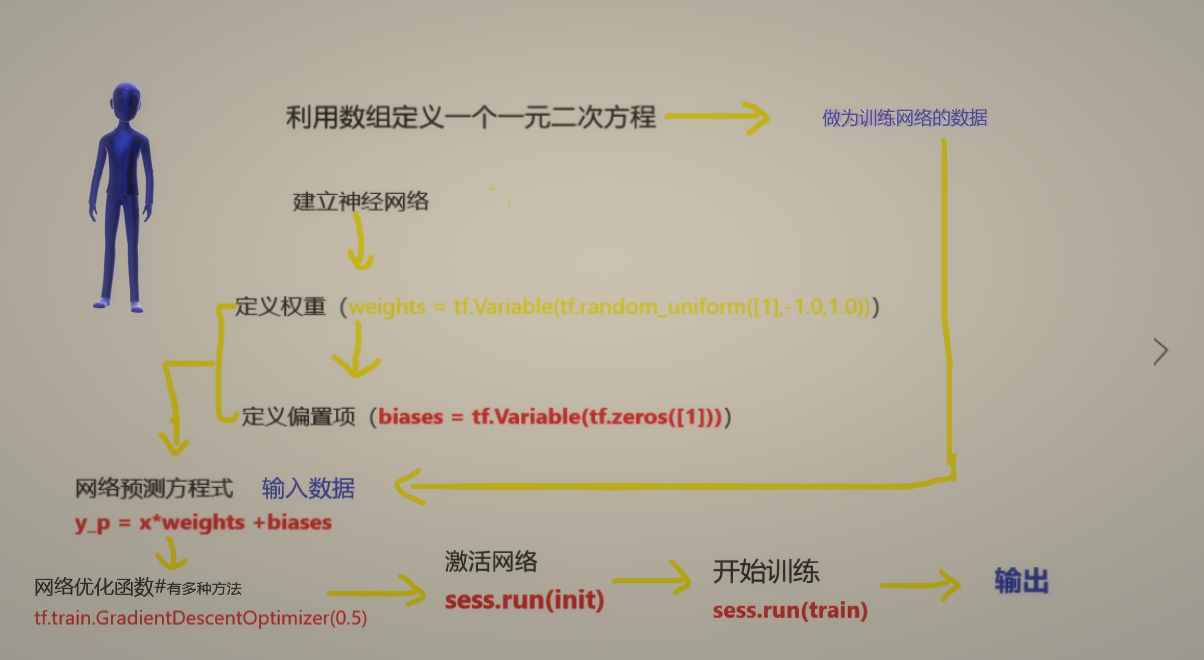# python从入门到放弃——tensorflow

1.杂乱无章的初步认识

1.经典例子零件检测

2.实战演练----利用网络寻找二元一次方程y = 5*x +3的参数。

（建议直接从目录二开始，一自己留作纪念）

# 一.自己学习tensorflow的来由

最近公司安排学习python，同时研究一下深度学习，人工智能识别，最后的目的就是实现利用机器学习自动完成影像的对别，识别房屋，自动生成地形图等。总之走在时尚最前沿了，说干就干，但是入门的路上坑真的太多，写在这里算是提醒，总结，也可以作为后的某一天的回味吧。

首先感谢一下我的领导，提供了学习环境和机会，感激。

from PIL import Image
import pytesseract
#text = pytesseract.image_to_string(Image.open("1.jpg"),lang="chi_sim")
image = Image.open("1.jpg")
text = pytesseract.image_to_string(image,lang="chi_sim")
print(text)
软件安装注意的就是tess中要设置中文，然后是环境变量，环境变量真的重要，现在才明白控制台为什么

TensorFlow的安装方式很多，要有两个软件protocol buffer和bazel。安装一开始使用docker，费尽周折，不过这个软件可以留着使用了。然后用pip安装 直接输入：

pip install tensorflow

TensorFlow使用

## 1.杂乱无章的初步认识

import tensorflow as tf #使用tf代替模块名称

graph （图表） 查看计算图 创建计算图

tf.get_default_graph 获取计算图

g1 = tf.graph

with g1.as_default():#定义g1中的变量V

v = tf.get_variable(

"v",initializer = tf.zeros_initializer(shape=))#初始化值为0

#读取

with tf.Session(graph=g1) as sess:

tf.initializer_all_variable().run()

with tf.variable_scope("",reuse=ture) :

print(sess.run(tf.get_variable("v")))

--------------------------------------------分割线---2018.10.19-------------------------------------------------------------------------------

# 二.学习使用tensorflow

## 1.经典例子零件检测import tensorflow as tf
#,stddev=1,seed=1))#两个输入,三个节点,形成二行三列的矩阵,stddve,标准差,seed随机种子
w1 = tf.Variable(tf.random_normal([2,3]))
#三个节点,一个输出定义一个三行一列的矩阵.
w2 = tf.Variable(tf.random_normal([3,1]))
#x就是输入层的二维矩阵
x = tf.constant([[0.7,0.5]])
#tf.matmul()计算矩阵乘法
a = tf.matmul(x,w1)#输入层到隐藏层的计算
y = tf.matmul(a,w2)#隐藏层到输出层的计算

with tf.Session() as sess:#定义会话,利用上下文管理器实现资源回收
sess.run(w1.initializer)#变量需要初始化,x为常量不需要
sess.run(w2.initializer)
print(sess.run(y))#利用此方法得到y的取值

## 2.实战演练----利用网络寻找二元一次方程y = 5*x +3的参数。

import tensorflow as tf
import numpy as np
# hello = tf.constant('Hello, TensorFlow!')
# sess = tf.Session()
# print(sess.run(hello))
x = np.random.rand(100).astype(np.float32)
y = x*5 + 3
weights = tf.Variable(tf.random_uniform(,-1.0,1.0))#定义权重变量
biases = tf.Variable(tf.zeros())#偏置项
y_p = x*weights + biases #预测方程
loss =tf.reduce_mean(tf.square(y_p-y))#误差
train = best.minimize(loss)#每一步都让loss减小
init = tf.initialize_all_variables()#初始化网络
sess = tf.Session()#要开始
sess.run(init)#激活网络
for step in range(200):
sess.run(train)#执行训练
if step%20 == 0:
print(step,sess.run(loss),sess.run(weights),sess.run(biases))#sess.run能输出网络0 2.2383208 [2.9448485] [5.338428]
20 0.034321316 [4.363446] [3.3206532]
40 0.0020912993 [4.8428693] [3.079152]
60 0.00012742727 [4.961213] [3.0195382]
80 7.764437e-06 [4.9904256] [3.004823]
100 4.7323243e-07 [4.9976363] [3.0011907]
120 2.885422e-08 [4.9994164] [3.000294]
140 1.7672704e-09 [4.9998555] [3.0000727]
160 1.0483973e-10 [4.9999647] [3.0000176]
180 7.1173644e-12 [4.999991] [3.0000045]